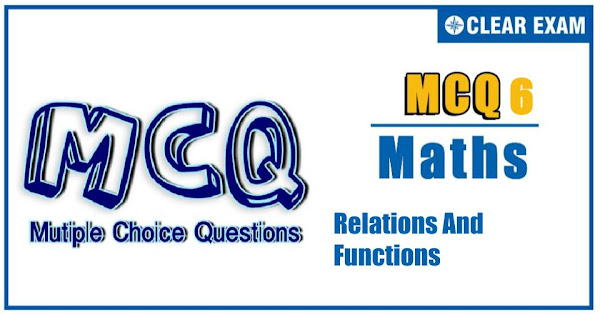## RELATIONS AND FUNCTIONS Quiz-6

In mathematics, a function can be defined as a rule that relates every element in one set, called the domain, to exactly one element in another set, called the range. For example, y = x + 3 and y = x2 – 1 are functions because every x-value produces a different y-value. A relation is any set of ordered-pair numbers..

Q1. The domain of the function f(x)=√(x^2-〖[x]〗^2 ), where [x]= the greatest integer less than or equal to x, is
•  R
•  [0,+∞)
•  (-∞,0]
•  None of these
Solution
(d) x^2-〖[x]〗^2≥0⇒x^2≥〖[x]〗^2 This is true for all positive values of x and all negative integer x

Q2.If the period of cos⁡(sin⁡(nx) )/tan⁡(x⁄n) ,n∈N, is 6π, then n is equal to
•  3
•  2
•  6
•  1
Solution
(c) The period of cos⁡(sin⁡nx ) is π/n and the period of tan⁡(x/n) is π n Thus, 6π=LCM(π/n,πn) By checking for the different values of n,n=6

Q3.  Let R be the set o real numbers. If R→R is a function defined by f(x)=x^2, then f is
•   Injective but not surjective
•  Surjective but not injective
•  Bijective
•  None of these
Solution
(d) f(x)=x^2 is many-one as f(1)=f(-1)=1. Also f is into, as the range of function is [0,∞) which is subset of R (co-domin). ∴f is neither injective nor surjective.

Q4. The range of f(x)=[sin⁡x+[cos⁡x+[tan⁡x+[sec⁡x ]]]],x∈(0,π⁄4), where [.] denotes the greatest integer function ≤x, is
•  {0, 1}
•  {-1, 0, 1}
•  {1}
•  None of these
Solution
(c) Given f(x)=[sin⁡x+[cos⁡x+[tan⁡x+[sec⁡x ]]]] =[sin⁡〖+p〗 ],where p=[cos⁡x+[tan⁡x+[sec⁡x ]]] =[sin⁡x ]+p, (as p is integer) =[sin⁡x ]+[cos⁡x+[tan⁡x+[sec⁡x ]]] =[sin⁡x ]+[cos⁡x ]+[tan⁡x ]+[sec⁡x ] Now, for x∈(0,π⁄4),sin⁡x∈(0,1/√2),cos⁡x∈(1/√2,1),tan⁡x∈(0,1),sec⁡x∈(1,√2) ⇒[sin⁡x ]=0,[cos⁡x ]=0,[tan⁡x ]=0 and [sec⁡x ]=1 ⇒ The range of f(x) is 1

Q5. Let f:R→[0,π/2) defined by f(x)=tan^(-1)⁡〖(x^2+x+a)〗, then the set of values of a for which f is onto is
•  [0,∞)
•  [2,1]
•  [1/4,∞)
•  None of these
Solution
(c) Since co-domain =[0,π/2) ∴ for f to be onto, the range =[0,π/2) This is possible only when x^2+x+a≥0∀x∈R ∴1^2-4a≤0⇒a≥1/4

Q6. The domain of the function f(x)=1/√(4x-|x^2-10x+9| ) is
•  (7-√40,7+√40)
•  (0,7+√40)
• (7-√40,∞)
•  None of these
Solution
(d) f(x)=1/√(4x-|x^2-10x+9| ) For f(x) to be defined |x^2-10x+9|

Q7. Range of the function f(x)=(x^2+x+2)/(x^2+x+1);x∈R is
•  (1,∞)
•  (1, 11/7)
•  [1, 7/3]
•  (1, 7/5)
Solution
(c) Let y=(x^2+x+2)/(x^2+x+1) ⇒x^2 (y-1)+x(y-1)+(y-2)=0,∀ x∈R Now, D≥0 ⇒(y-1)^2-4(y-1)(y-2)≥0 ⇒ (y-1){(y-1)-4(y-2)}≥0 ⇒ (y-1)(-3y+7)≥0 ⇒ 1≤y≤7/3

Q8. The function f:R→R is defined by f(x)=cos^2⁡x+sin^4⁡x for x∈R, then the range of f(x) is
•  (3/4,1]
•  T[3/4,1)
•  [3/4,1]
•  (3/4,1)
Solution
(c) y=f(x)=cos^2⁡x+sin^4⁡x ⇒ y=f(x)=cos^2⁡x+sin^2⁡x (1-cos^2⁡x ) ⇒y=cos^2⁡x+sin^2⁡x-sin^2⁡x cos^2⁡x ⇒y=1-sin^2⁡x cos^2⁡x ⇒y=1-〖1/4 sin^2〗⁡2x ∴3/4≤f(x)≤1 (∵0≤sin^2⁡2x≤1) ⇒ f(x)∈[3⁄4,1]

Q9. f(x)=cos⁡x/([2x/π]+1/2), where x is not an integral multiple of π and [.] denotes the greatest integer function is
•  An odd function
•  Even function
•  Neither odd nor even
•  None of these
Solution
(a) f(-x)=cos⁡〖(-x)〗/([-2x/π]+1/2)=cos⁡x/(-1-[2x/π]+1/2) (as x is not an integral multiple of π) ⇒ f(-x)=-cos⁡x/([2x/π]+1/2)=-f(x) ⇒f(x) is an odd function.

Q10. The number of solutions of the equation [y+[y]]=2 cos⁡x, where y=1/3 [sin⁡x+[sin⁡x+[sin⁡x ]]] (where [.] denotes the greatest integer function) is
•  4
•  2
•  3
• 53
Solution
60 (d) [y+[y]]=2 cos⁡x ⇒[y]+[y]=2 cos⁡x (∵[x+n]=[x]+n if n∈I) ⇒2[y]=2 cos⁡x⇒[y]=cos⁡x (1) Also y=1/3 [sin⁡x+[sin⁡x+[sin⁡x ]]] =1/3 (3[sin⁡x ]) =[sin⁡x] (2) From (1) and (2) [[sin⁡x ]]=cos⁡x ⇒[sin⁡x ]=cos⁡x The number of solutions is 0#### Written by: AUTHORNAME

AUTHORDESCRIPTION## Want to know more

Please fill in the details below:

## Latest NEET Articles\$type=three\$c=3\$author=hide\$comment=hide\$rm=hide\$date=hide\$snippet=hide

Name

ltr
item
BEST NEET COACHING CENTER | BEST IIT JEE COACHING INSTITUTE | BEST NEET & IIT JEE COACHING: Relations and Functions-Quiz-6
Relations and Functions-Quiz-6
https://1.bp.blogspot.com/-w2qwJJYqMGE/YN88tSmu1fI/AAAAAAAAI_I/O-xt2dRqOMkg5bhePDf15RgPRYcNwQjiwCLcBGAsYHQ/s600/Quiz%2BImage%2B20%2B%25285%2529.jpg
https://1.bp.blogspot.com/-w2qwJJYqMGE/YN88tSmu1fI/AAAAAAAAI_I/O-xt2dRqOMkg5bhePDf15RgPRYcNwQjiwCLcBGAsYHQ/s72-c/Quiz%2BImage%2B20%2B%25285%2529.jpg
BEST NEET COACHING CENTER | BEST IIT JEE COACHING INSTITUTE | BEST NEET & IIT JEE COACHING
https://www.cleariitmedical.com/2021/07/relations-and-functions-quiz-6.html
https://www.cleariitmedical.com/
https://www.cleariitmedical.com/
https://www.cleariitmedical.com/2021/07/relations-and-functions-quiz-6.html
true
7783647550433378923
UTF-8

STAY CONNECTED